Ex 9.3

Chapter 9 Class 9 - Areas of Parallelograms and Triangles (Deleted)
Serial order wise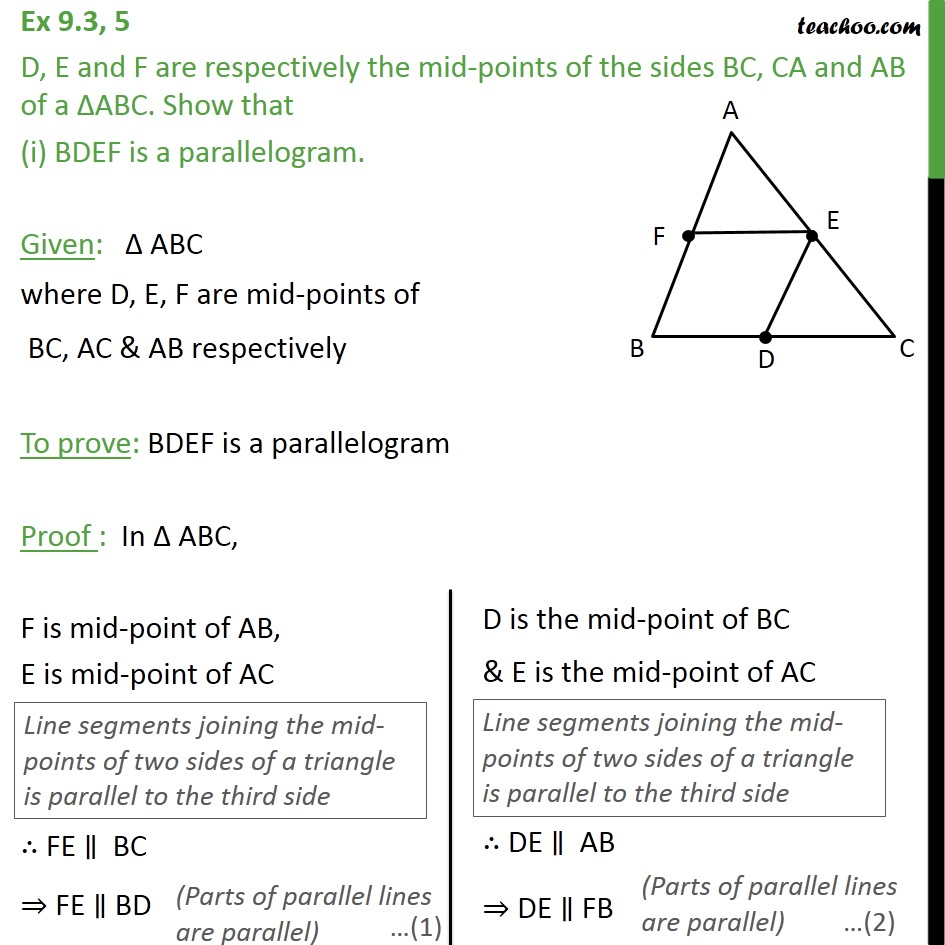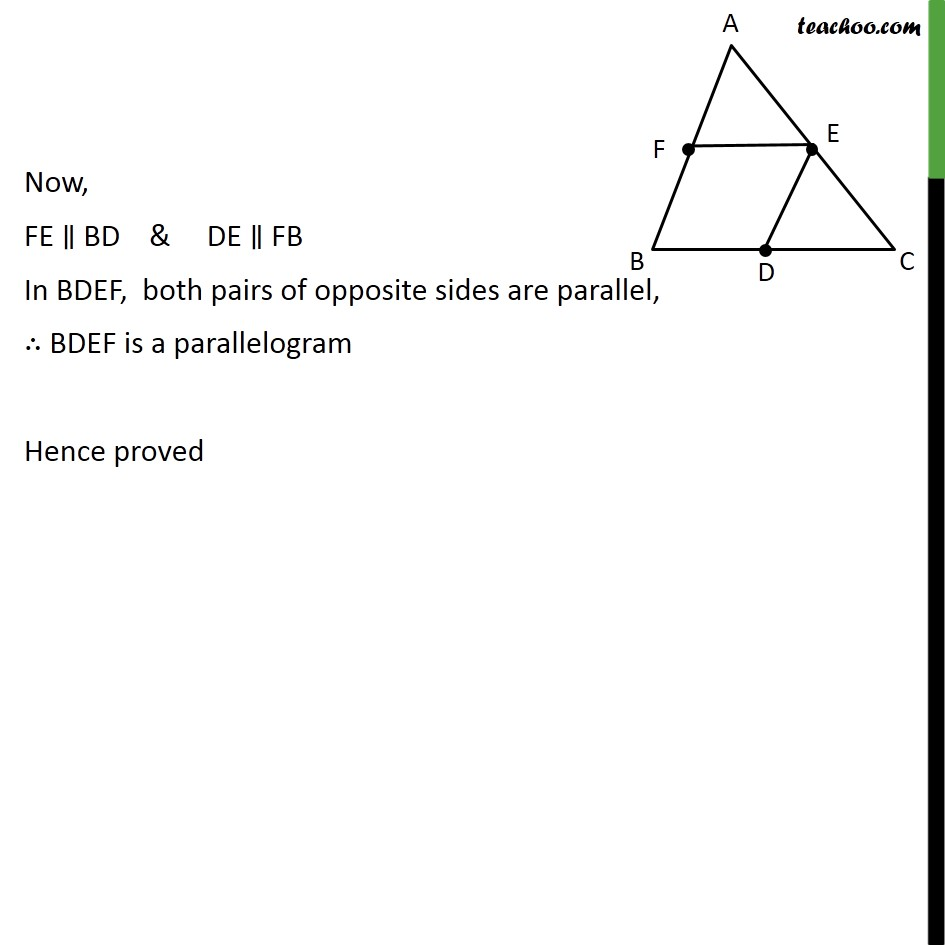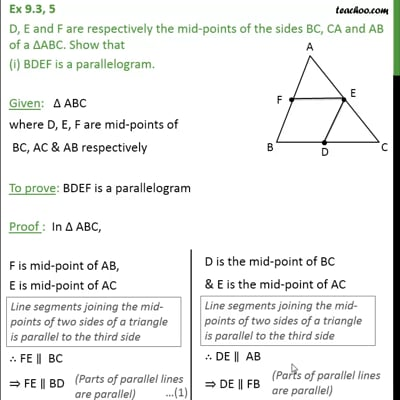This video is only available for Teachoo black users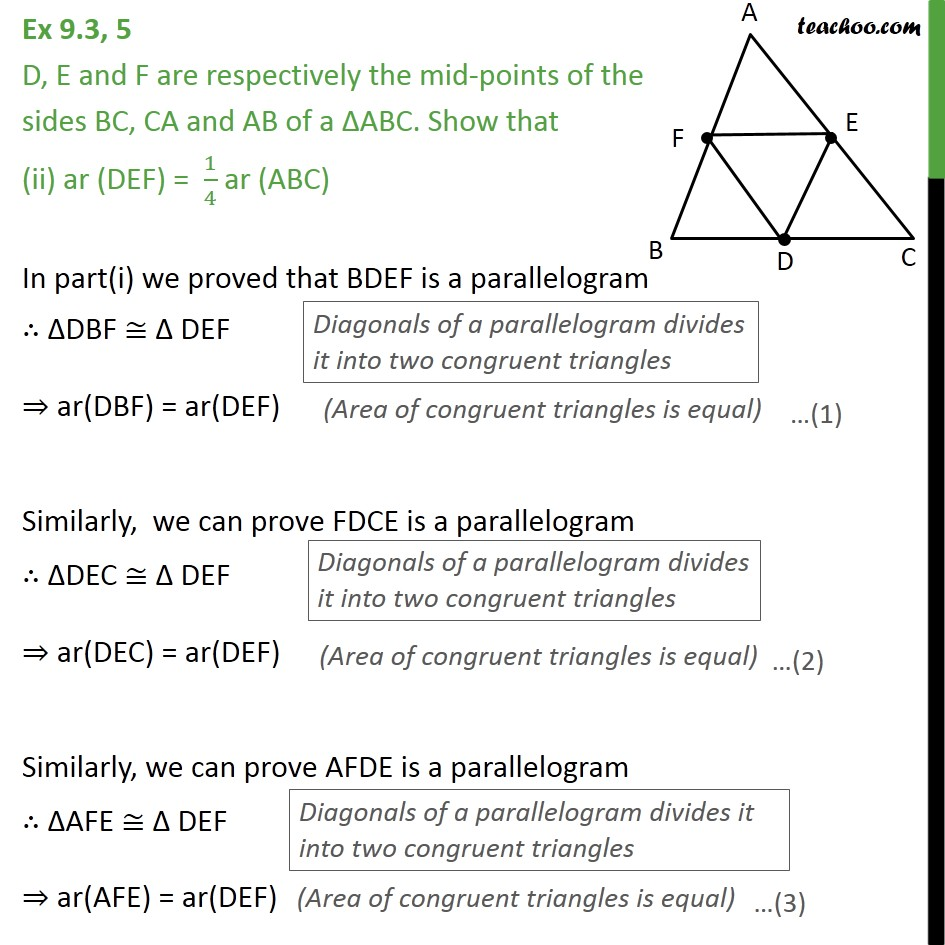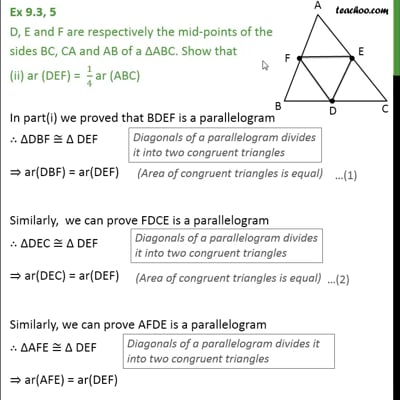This video is only available for Teachoo black users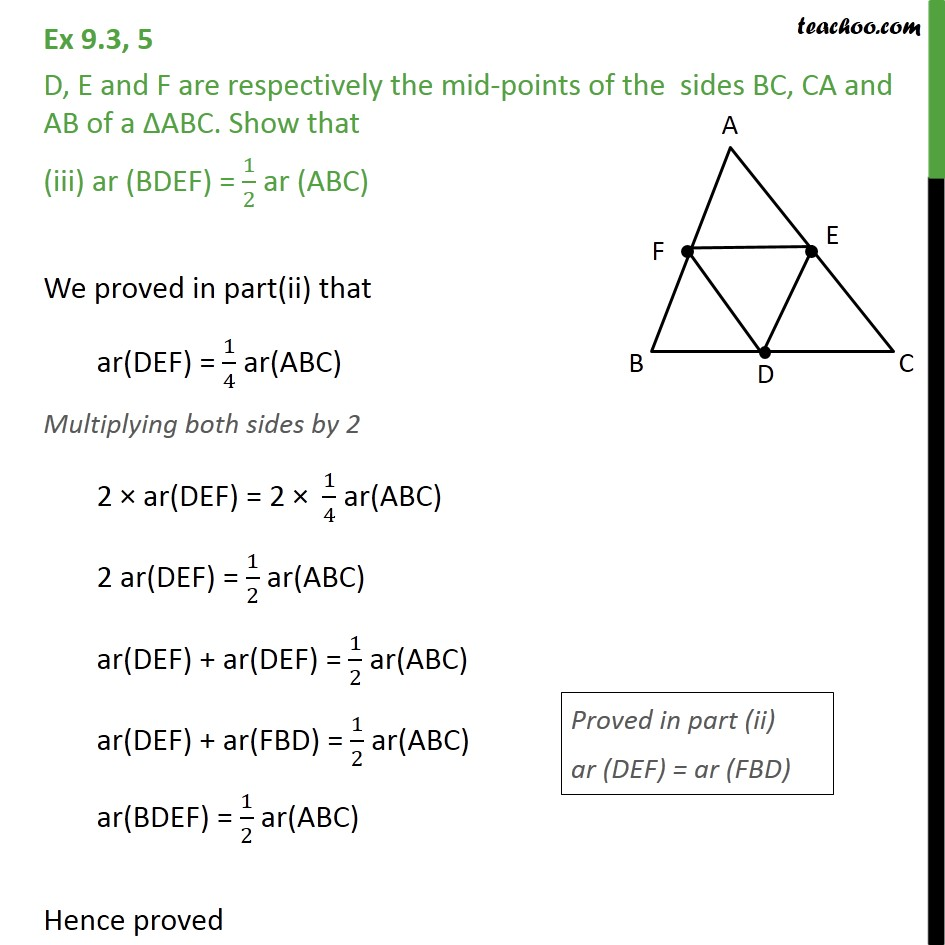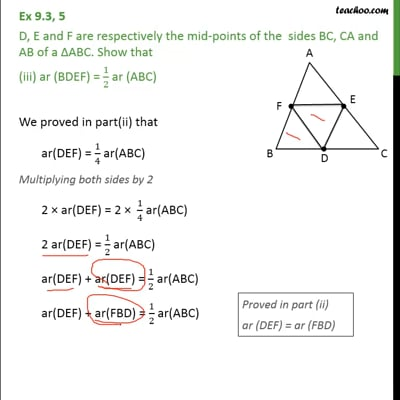This video is only available for Teachoo black users

### Transcript

Ex 9.3, 5 D, E and F are respectively the mid-points of the sides BC, CA and AB of a ABC. Show that (i) BDEF is a parallelogram. Given: ABC where D, E, F are mid-points of BC, AC & AB respectively To prove: BDEF is a parallelogram Proof : In ABC, F is mid-point of AB, E is mid-point of AC FE BC FE BD Now, FE BD & DE FB In BDEF, both pairs of opposite sides are parallel, BDEF is a parallelogram Hence proved Ex 9.3, 5 D, E and F are respectively the mid-points of the sides BC, CA and AB of a ABC. Show that (ii) ar (DEF) = 1/4 ar (ABC) In part(i) we proved that BDEF is a parallelogram DBF DEF ar(DBF) = ar(DEF) Similarly, we can prove FDCE is a parallelogram DEC DEF ar(DEC) = ar(DEF) Similarly, we can prove AFDE is a parallelogram AFE DEF ar(AFE) = ar(DEF) From (1),(2) & (3) ar(FBD) = ar(DEC) = ar(AFE) = ar(DEF) Now ar(FBD) + ar(DEC) + ar(AFE) + ar(DEF) = ar(ABC) ar(DEF) + ar(DEF) + ar(DEF) + ar(DEF) = ar(ABC) 4ar(DEF) = ar(ABC) ar (DEF) = 1/4 ar (ABC) Hence proved Ex 9.3, 5 D, E and F are respectively the mid-points of the sides BC, CA and AB of a ABC. Show that (iii) ar (BDEF) = 1/2 ar (ABC) We proved in part(ii) that ar(DEF) = 1/4 ar(ABC) Multiplying both sides by 2 2 ar(DEF) = 2 1/4 ar(ABC) 2 ar(DEF) = 1/2 ar(ABC) ar(DEF) + ar(DEF) = 1/2 ar(ABC) ar(DEF) + ar(FBD) = 1/2 ar(ABC) ar(BDEF) = 1/2 ar(ABC) Hence proved